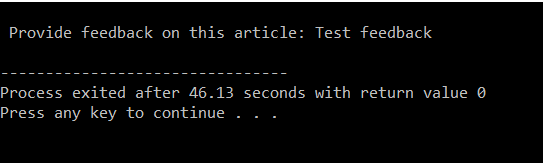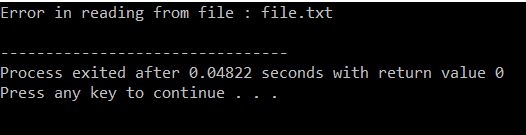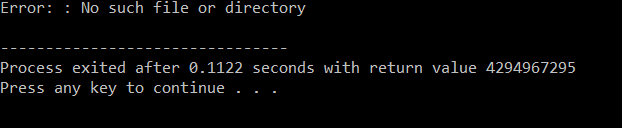Related Articles

# Error handling during file operations in C/C++

• Difficulty Level : Basic
• Last Updated : 13 Jan, 2021

It is quite common that errors may occur while reading data from a file in C++ or writing data to a file. For example, an error may arise due to the following:

• When trying to read a file beyond indicator.
• When trying to read a file that does not exist.
• When trying to use a file that has not been opened.
• When trying to use a file in an appropriate mode i.e., writing data to a file that has been opened for reading.
• When writing to a file that is write-protected i.e., trying to write to a read-only file.

Failure to check for errors then the program may behave abnormally therefore an unchecked error may result in premature termination for the program or incorrect output.

Below are some Error handling functions during file operations in C/C++:

### ferror():

In C/C++, the library function ferror() is used to check for the error in the stream. Its prototype is written as:

int ferror (FILE *stream);

The ferror() function checks for any error in the stream. It returns a value zero if no error has occurred and a non-zero value if there is an error. The error indication will last until the file is closed unless it is cleared by the clearerr() function.

Below is the program to implement the use of ferror():

## C

 `// C program to illustrate the``// use of ferror()``#include ``#include `` ` `// Driver Code``int` `main()``{``    ``FILE``* fp;`` ` `    ``// If a file is opened which does``    ``// not exist, then it will be an``    ``// error and corresponding errno``    ``// value will be set``    ``char` `feedback;`` ` `    ``int` `i;``    ``fp = ``fopen``(``"GeeksForGeeks.TXT"``, ``"w"``);`` ` `    ``if` `(fp == NULL) {``        ``printf``(``"\n The file could "``               ``"not be opened"``);``        ``exit``(1);``    ``}`` ` `    ``printf``(``"\n Provide feedback on "``           ``"this article: "``);``    ``fgets``(feedback, 100, stdin);`` ` `    ``for` `(i = 0; i < feedback[i]; i++)``        ``fputc``(feedback[i], fp);`` ` `    ``// Error writing file``    ``if` `(``ferror``(fp)) {``        ``printf``(``"\n Error writing in file"``);``        ``exit``(1);``    ``}`` ` `    ``// Close the file pointer``    ``fclose``(fp);``}`

## C++

 `// C++ program to illustrate the``// use of ferror()``#include `` ` `// Driver Code``int` `main()``{``    ``FILE``* fp;`` ` `    ``// If a file is opened which does``    ``// not exist, then it will be an``    ``// error and corresponding errno``    ``// value will be set``    ``char` `feedback;`` ` `    ``int` `i;``    ``fp = ``fopen``(``"GeeksForGeeks.TXT"``, ``"w"``);`` ` `    ``if` `(fp == NULL) {``        ``printf``(``"\n The file could "``               ``"not be opened"``);``        ``exit``(1);``    ``}`` ` `    ``printf``(``"\n Provide feedback on "``           ``"this article: "``);``    ``fgets``(feedback, 100, stdin);`` ` `    ``for` `(i = 0; i < feedback[i]; i++)``        ``fputc``(feedback[i], fp);`` ` `    ``// Error writing file``    ``if` `(``ferror``(fp)) {``        ``printf``(``"\n Error writing in file"``);``        ``exit``(1);``    ``}`` ` `    ``// Close the file pointer``    ``fclose``(fp);``}`

Output:Explanation: After executing this code “Provide feedback on this article:“ will be displayed on the screen and after giving some feedback “Process exited after some seconds with return value 0″ will be displayed on the screen.

### clearerr():

The function clearerr() is used to clear the end-of-file and error indicators for the stream. Its prototype can be given as:

void clearerr(FILE *stream);

The clearerr() clears the error for the stream pointed by the stream. The function is used because error indicators are not automatically cleared. Once the error indicator for a specific stream is set, operations on the stream continue to return an error value until clearerr(), fseek(), fsetpos(), or rewind() is called.

Below is the program to implement the use of clearerr():

## C

 `// C program to illustrate the``// use of clearerr()``#include ``#include ``#include `` ` `// Driver Code``int` `main()``{``    ``FILE``* fp;``    ``char` `feedback;`` ` `    ``char` `c;`` ` `    ``fp = ``fopen``(``"file.txt"``, ``"w"``);`` ` `    ``c = ``fgetc``(fp);``    ``if` `(``ferror``(fp)) {``        ``printf``(``"Error in reading from"``               ``" file : file.txt\n"``);``    ``}``    ``clearerr``(fp);`` ` `    ``if` `(``ferror``(fp)) {``        ``printf``(``"Error in reading from "``               ``"file : file.txt\n"``);``    ``}`` ` `    ``// close the file``    ``fclose``(fp);``}`

## C++

 `// C++ program to illustrate the``// use of clearerr()``#include `` ` `// Driver Code``int` `main()``{``    ``FILE``* fp;``    ``char` `feedback;`` ` `    ``char` `c;`` ` `    ``fp = ``fopen``(``"file.txt"``, ``"w"``);`` ` `    ``c = ``fgetc``(fp);``    ``if` `(``ferror``(fp)) {``        ``printf``(``"Error in reading from"``               ``" file : file.txt\n"``);``    ``}``    ``clearerr``(fp);`` ` `    ``if` `(``ferror``(fp)) {``        ``printf``(``"Error in reading from "``               ``"file : file.txt\n"``);``    ``}`` ` `    ``// close the file``    ``fclose``(fp);``}`

Output:The function perror() stands for print error. In case of an error, the programmer can determine the type of error that has occurred using the perror() function. When perror() is called, then it displays a message describing the most recent error that occurred during a library function call or system call. Its prototype can be given as:

void perror (char*msg);

• The perror() takes one argument which points to an optional user-defined message the message is printed first followed by a colon and the implementation-defined message that describes the most recent error.
• If a call to perror() is made when no error has actually occurred then ‘No error’ will be displayed.
• The most important thing to remember is that a call to perror() and nothing is done to deal with the error condition, then it is entirely up to the program to take action. For example, the program may prompt the user to do something such as terminate the program.
• Usually, the program’s activities will be determined by checking the value of errno and the nature of the error.
• In order to use the external constant errno, you must include the header file ERRNO.H

Below is the program given below illustrates the use of perror(). Here, assume that the file “file.txt” does not exist.

## C

 `// C program to illustrate the``// use of perror()``#include ``#include ``#include `` ` `// Driver Code``int` `main()``{``    ``FILE``* fp;`` ` `    ``// First rename if there is any file``    ``rename``(``"file.txt"``, ``"newfile.txt"``);`` ` `    ``// Now try to open same file``    ``fp = ``fopen``(``"file.txt"``, ``"r"``);`` ` `    ``if` `(fp == NULL) {`` ` `        ``perror``(``"Error: "``);``        ``return` `(-1);``    ``}`` ` `    ``// Close the file pointer``    ``fclose``(fp);`` ` `    ``return` `(0);``}`

## C++

 `// C++ program to illustrate the``// use of perror()``#include ``#include `` ` `// Driver Code``int` `main()``{``    ``FILE``* fp;`` ` `    ``// First rename if there is any file``    ``rename``(``"file.txt"``, ``"newfile.txt"``);`` ` `    ``// Now try to open same file``    ``fp = ``fopen``(``"file.txt"``, ``"r"``);`` ` `    ``if` `(fp == NULL) {`` ` `        ``perror``(``"Error: "``);``        ``return` `(-1);``    ``}`` ` `    ``// Close the file pointer``    ``fclose``(fp);`` ` `    ``return` `(0);``}`

Output:Take a step-up from those “Hello World” programs. Learn to implement data structures like Heap, Stacks, Linked List and many more! Check out our Data Structures in C course to start learning today.

My Personal Notes arrow_drop_up